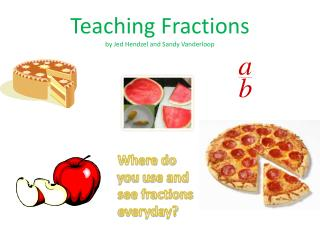DownloadDownload PresentationTeaching Fractions by Jed Hendzel and Sandy Vanderloop

# Teaching Fractions by Jed Hendzel and Sandy Vanderloop

Télécharger la présentation## Teaching Fractions by Jed Hendzel and Sandy Vanderloop

- - - - - - - - - - - - - - - - - - - - - - - - - - - E N D - - - - - - - - - - - - - - - - - - - - - - - - - - -
##### Presentation Transcript

1. Teaching Fractionsby Jed Hendzel and Sandy Vanderloop Where do you use and see fractions everyday?

2. Making Connections to Fractions • Fraction words • Whole – the entire part • Part – a piece or part of a whole unit • Fraction – one or more parts of a whole or unit • Halves - what fraction of the letters are green? 3/6 or 1/2 • Thirds - what fraction of the letters are red? 2/6 or 1/3 The numerator tells you how many parts we are talking about, can this number ever be zero? The denominator tells you how many parts the whole is divided into

3. Part-WholeThe whole has been partitioned into equal parts • Region – region is a whole and parts are congruent • Length – any unit of length can be partitioned into fractional parts of equal length • Set – uses a set of objects as a whole • Area - parts must be equal in area but not necessarily congruent

4. RegionRegion is a whole and parts are congruent http://www.globalclassroom.org

5. Length Any unit of length can be partitioned into fractional parts of equal length How many 1/12’s are in a whole? How many 1/8’s are in a whole http://bigshanty.blogs.com/Ashburn/

6. SetUses a set of objects as a whole Which part of the set has single digits? Which part of the set is striped? 9/15 or 3/5 Three-fifths 7/15 Seven-fifths Which part of the set uses the number 1? Which part of the set is black? 7/15 1/15 Seven-fifteenths One -fifteenths

7. AreaParts must be equal in area but not necessarily congruent If Jed ate 1 slice of the apple what fraction did he eat? What fraction of the apple is left? How would you write that?

8. Quotient Partitioning – sharing equally If we have 12 carrot sticks how would you share them equally with 4 friends?

9. Ratio 1 teacher ______ 5 children One-fifth

10. Equivalent Fractions 1/2 is equal to 5/10 1/3 is equal to 3/9 http://ssrsbstaff.ednet.ns.ca/jcroft2/fractions.htm

11. Comparing and Ordering Fractions Are these fractions equal? 7/16 < 3/4 3/16 < 5/8

12. Mixed Fractions 1 1/2 1 2/8 wholes and parts

13. Improper Fractions http://spmath81708.blogspot.com http://www.instructables.com

14. Adding and Subtracting Fractions with like denominators http://www.inspiration.com/Kidspiration-math-examples

15. Adding Fractions with unlike denominators http://www.helpingwithmath.com

16. Subtracting Fractions with unlike denominators To subtract the fractions, we need to make their denominators match. visualfractions.com

17. Multiplying and Dividing Fractions Multiply the numerators together and multiply the denominators together

18. Dividing Fractions http://www.visualfractions.com/divide.htm